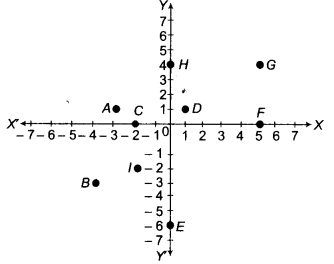# From the given figure, write

From the given figure, write(i)|the ordinate of the points A and C.
(ii)the point identified by the coordinates (1,1).
(iii)the perpendicular distance of the point G from the X-axis.
(iv)the coordinates of the points B and F.
(v)the perpendicular distance of the point I from the Y-axis.
(vi)the points whose perpendicular distance from Y-axis is 2 units.

(i) Coordinates of points A and C are (-3, 1) and (-2, 0), respectively.
Hence, ordinate of points A and C are 1 and 0.
(ii) (1,1) represents the point D.
(iii) Coordinates of point G is (5, 4).
Hence, the perpendicular distance of the point G from the X-axis is 5 units.
(iv) Coordinates of points B and F are (-4, - 3) and (5,0) respectively.
(v) Coordinates of point / is (-2, -2).
Hence, the perpendicular distance of the point l from the Y-axis is -2 units.
(vi) There are two points C and / whose distance from Y-axis is 2 units.